Updating search results...

# 48 Results

View
Selected filters:
• NC.Math.3.NF.3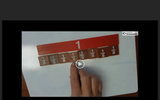Unrestricted Use
CC BY
Rating
0.0 stars
Overview:

2ND VIDEO IN A THREE PART SERIES This video addresses standard 3.NF.3 Fractions with the same numerator and denominator equals one whole.

Subject:
Mathematics
Material Type:
Interactive
Teaching/Learning Strategy
Author:
Shannon Curry
Susie Grace
08/20/2019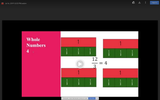Unrestricted Use
CC BY
Rating
0.0 stars
Overview:

3rd VIDEO IN A THREE PART SERIES: This video addresses standard 3.NF.3 Fractions can be decomposed to equal whole numbers and whole numbers can be decomposed to equal fractional amounts.

Subject:
Mathematics
Material Type:
Curriculum
Teaching/Learning Strategy
Author:
Shannon Curry
Susie Grace
08/20/2019Rating
3.0 stars
Overview:

In this task, pairs of students compare two fractions on a card and determine if they are equivalent and, if not, which is larger.

Subject:
Mathematics
Material Type:
Activity/Lab
Provider:
Illustrative Mathematics
Author:
Illustrative Mathematics
02/26/2019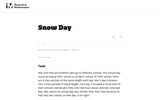Unrestricted Use
CC BY
Rating
5.0 stars
Overview:

This is a task from the Illustrative Mathematics website that is one part of a complete illustration of the standard to which it is aligned. Each task has at least one solution and some commentary that addresses important asects of the task and its potential use. Here are the first few lines of the commentary for this task: Alec and Felix are brothers who go to different schools. The school day is just as long at Felix' school as at Alec's school. At Felix' school, there a...

Subject:
Mathematics
Material Type:
Activity/Lab
Provider:
Illustrative Mathematics
Provider Set:
Illustrative Mathematics
Author:
Illustrative Mathematics
05/17/2013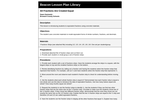Rating
5.0 stars
Overview:

In this lesson, students are introduced to equivalent fractions using fraction strips. This lesson focuses on 1/2, 1/3, 1/4, 1/5, 1/6, 1/8.

Subject:
Mathematics
Material Type:
Activity/Lab
Provider:
Beacon Learning Center
Author:
Dawn Dantowitz
02/26/2019
RemixUnrestricted Use
CC BY
Rating
0.0 stars
Overview:

Students can apply their knowledge of fractions and equivalent fractions to a real life situation....cooking!
Students are to complete work using manipulatives, and/or paper and pencil to draw out their work.

Subject:
Mathematics
Material Type:
Homework/Assignment
Self Assessment
Author:
ERIN WOLFHOPE
04/21/2020Unrestricted Use
CC BY
Rating
0.0 stars
Overview:

Students can apply their knowledge of fractions and equivalent fractions to a real life situation....cooking!
Students are to complete work using manipulatives, and/or paper and pencil to draw out their work.

Subject:
Mathematics
Material Type:
Homework/Assignment
Self Assessment
Author:
WENDY WILKINS
11/06/2019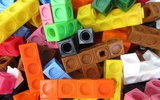Conditional Remix & Share Permitted
CC BY-NC-SA
Rating
0.0 stars
Overview:

In this lesson students create fraction strips to represent fractions and explore equivalent fractions.

Subject:
Mathematics
Material Type:
Lesson Plan
Author:
Drew Polly
07/12/2020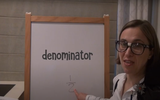Unrestricted Use
CC BY
Rating
0.0 stars
Overview:

This lesson is Day 10 in a series of 12 lessons around fraction equivalences and comparisons.

This video lesson serves as a review of equpartitioning in order to determine the fractional part of an area or length. This is a necessary foundational skill for comparing and finding equivalent fractions.

Subject:
Mathematics
Material Type:
Activity/Lab
Interactive
Lesson
Simulation
Author:
Dawne Coker
06/25/2020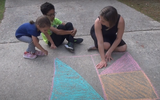Unrestricted Use
CC BY
Rating
0.0 stars
Overview:

This lesson is Day 11 in a series of 12 lessons around fraction equivalences and comparisons.

This video lesson reviews parts of a whole, comparing fractions, and equivalent fractions. No materials are needed.

Subject:
Mathematics
Material Type:
Activity/Lab
Interactive
Lesson
Simulation
Author:
Dawne Coker
06/25/2020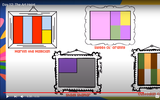Unrestricted Use
CC BY
Rating
0.0 stars
Overview:

This lesson is Day 12 in a series of 12 lessons around fraction equivalences and comparisons.

Use your math skills to help detectives solve the crime. This video lesson reviews fractions of an area, fractions on a number line, and equivalent fractions. No materials are required.

Subject:
Mathematics
Material Type:
Activity/Lab
Interactive
Lesson
Simulation
Author:
Dawne Coker
06/25/2020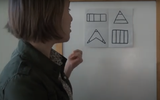Unrestricted Use
Public Domain
Rating
0.0 stars
Overview:

This lesson is Day 1 in a series of 12 lessons around fraction equivalences and comparisons.

This video lesson encourages students to reason about and visualize fractions. The lesson focuses on the ideas that fractions are fair shares and that fractions can be compared. This lesson assumes that students have some initial experiences with fractions.

Subject:
Mathematics
Material Type:
Interactive
Lesson
Presentation
Simulation
Author:
Dawne Coker
06/25/2020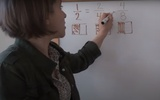Unrestricted Use
Public Domain
Rating
0.0 stars
Overview:

This lesson is Day 2 in a series of 12 lessons around fraction equivalences and comparisons.

This video lesson uses paper folding to introduce the concept of equivalent fractions. To complete this lesson, make sure you have a square piece of paper (any size) and a crayon

Subject:
Mathematics
Material Type:
Activity/Lab
Interactive
Lesson
Simulation
Author:
Dawne Coker
06/25/2020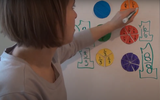Unrestricted Use
CC BY
Rating
0.0 stars
Overview:

This lesson is Day 2 in a series of 12 lessons around fraction equivalences and comparisons.

This video lesson introduces the idea that fractions can be used to represent 1 whole. To engage in this lesson, students may use virtual circle models from Toy Theater (https://toytheater.com/fraction-circles/)

Subject:
Mathematics
Material Type:
Activity/Lab
Interactive
Lesson
Simulation
Author:
Dawne Coker
06/25/2020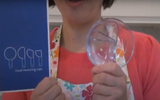Unrestricted Use
CC BY
Rating
0.0 stars
Overview:

This lesson is Day 4 in a series of 12 lessons around fraction equivalences and comparisons.

This video lesson looks at how we use fractions in the kitchen. Students are challenged to use circle models to find equivalent fractions for a recipe. Students may use virtual circe models from the Toy Theater (https://toytheater.com/fraction-circles/).

Subject:
Mathematics
Material Type:
Activity/Lab
Interactive
Lesson
Simulation
Author:
Dawne Coker
06/25/2020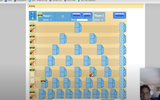Unrestricted Use
CC BY
Rating
0.0 stars
Overview:

This lesson is Day 2 in a series of 12 lessons around fraction equivalences and comparisons.

This game may be played with one or two people. It reviews fractions on a number line, adding fraction with like denominators, and equivalent fractions. For an extra challenge, you can even break up your moves across multiple tracks (e.g., if your card reads 1/2, you could move 1/4 and 2/8).

https://www.nctm.org/Classroom-Resources/Illuminations/Interactives/Playing-Fraction-Track/

Subject:
Mathematics
Material Type:
Activity/Lab
Interactive
Lesson
Simulation
Author:
Dawne Coker
06/25/2020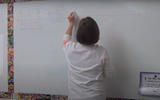Unrestricted Use
CC BY
Rating
0.0 stars
Overview:

This lesson is Day 9 in a series of 12 lessons around fraction equivalences and comparisons.

This video lesson introduces students to fractions beyond 1 using a choral counting routine. Students connect the fractions to points on a number line and bar models. For this lesson, students need a piece of blank paper and a pencil.

Subject:
Mathematics
Material Type:
Activity/Lab
Interactive
Lesson
Simulation
Author:
Dawne Coker
06/25/2020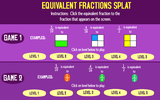Rating
0.0 stars
Overview:

In this interactive math game, students will click on the fraction that is equivalent to the fraction that appears on the screen. They can choose fast or slow mode. Once students complete the level they can choose another level to play.

Subject:
Mathematics
Material Type:
Interactive
Provider:
Sheppard Software
02/26/2019
RemixConditional Remix & Share Permitted
CC BY-NC-SA
Rating
0.0 stars
Overview:

Generate simple equivalent fractions by using visual fraction models and the number line.This lesson is adapted from a lesson created by Engage NY: https://www.engageny.org/resource/grade-3-mathematics-module-5-topic-e-lesson-22/file/35446The lesson image is Quarters by luca fruzza from the Noun Project.

Subject:
Mathematics
Material Type:
Lesson
Author:
ERIN WOLFHOPE
BRANDY MOODY
04/23/2020Conditional Remix & Share Permitted
CC BY-NC-SA
Rating
5.0 stars
Overview:

Generate simple equivalent fractions by using visual fraction models and the number line.This lesson is adapted from a lesson created by Engage NY: https://www.engageny.org/resource/grade-3-mathematics-module-5-topic-e-lesson-22/file/35446The lesson image is Quarters by luca fruzza from the Noun Project.

Subject:
Mathematics
Material Type:
Lesson
Author:
ERIN WOLFHOPE